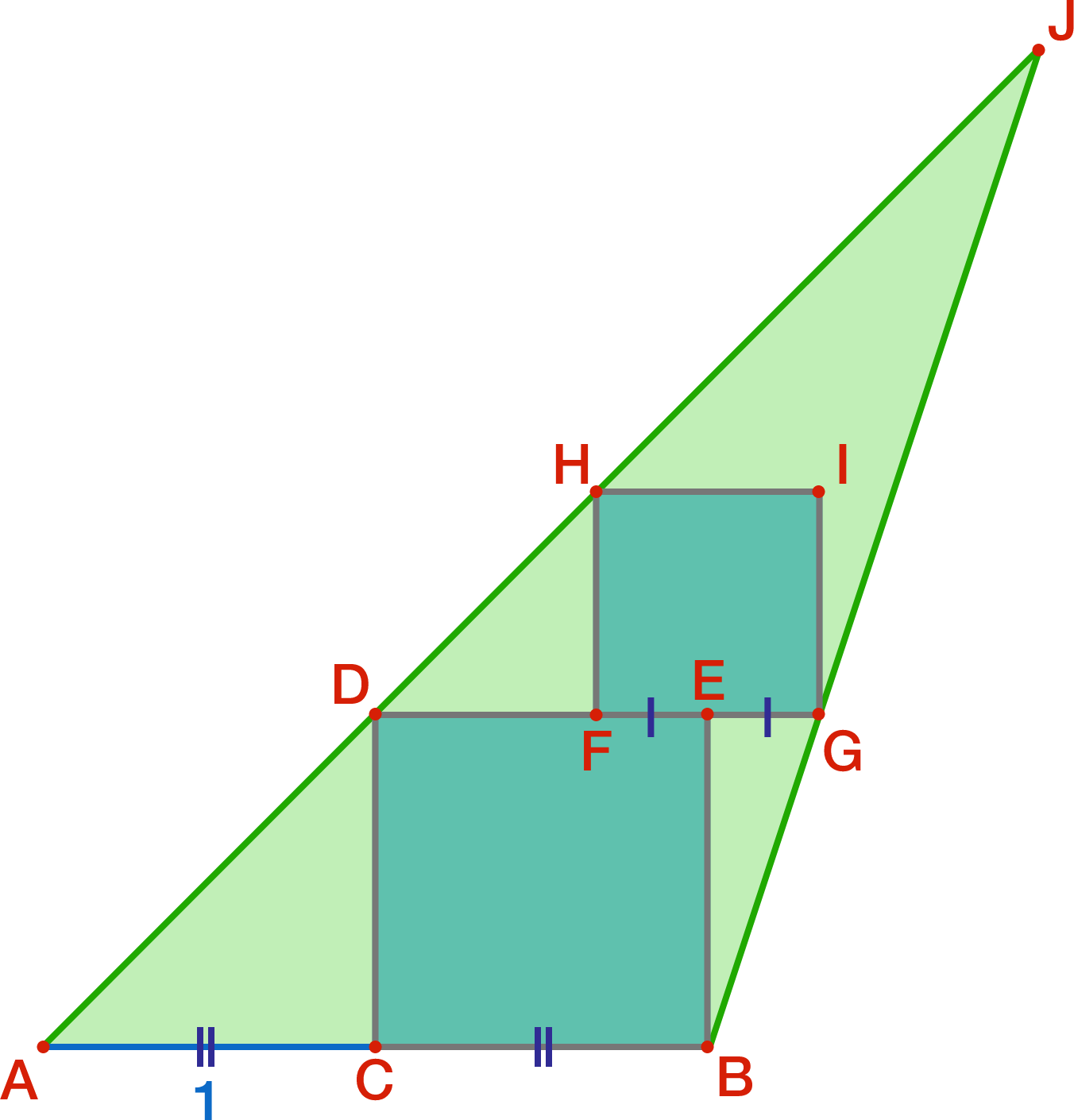# Is the information sufficient?

Geometry Level 4In the diagram, $AC=1$, where $C$ is the midpoint of side $AB$ of triangle $ABJ$. Square $BCDE$ is drawn, where $D$ lies on side $AJ$ and $E$ is in the interior of the triangle. Square $FGIH$ is drawn, where $G$ lies on side $BJ$, $H$ lies on side $AJ$, $I$ is in the interior of the square, and $E$ is the midpoint of $FG$.

Find the area of triangle $ABJ$.

×

Problem Loading...

Note Loading...

Set Loading...